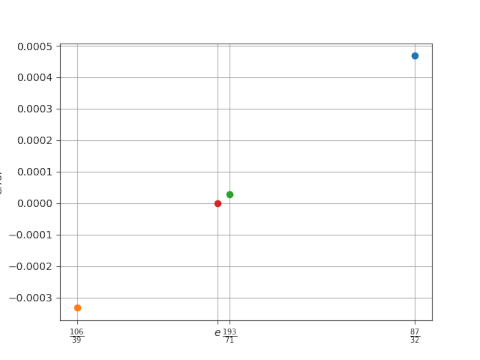# Mediant approximation trick

Suppose you are trying to approximate some number x and you’ve got it sandwiched between two rational numbers:

a/b < x < c/d.

Now you’d like a better approximation. What would you do?

The obvious approach would be to take the average of a/b and c/d. That’s fine, except it could be a fair amount of work if you’re doing this in your head.

I recently stumbled across an article  that suggested taking the mediant of the two fractions rather than the mean. The mediant of two fractions simply adds the numerators and denominators, the very thing you might have gotten your hand slapped for doing in elementary school. It’s not a valid way to add two fractions, but it is a valid way to get a new fraction between two fractions. That is,

a/b < (ac)/(bd) < c/d.

So the mediant of a lower bound and an upper bound is going to be a better approximation than either bound.

There are two advantages to using the mediant rather than the mean:

1. It’s easier to compute mentally.
2. It generally results in smaller numerators and denominators.

For example, suppose you know that 19/7 and 87/32 are both approximations to e, with

19/7 < e < 87/32.

Then (19 + 87)/(7 + 32) = 106/39 should be better than either of the previous approximations.

Here’s a little Python calculation to illustrate that this is the case.

```    >>> from math import exp
>>> e = exp(1)
>>> 19/7 - e
-0.003996114173330678
>>> 87/32 - e
0.0004681715409549092
>>> 106/39 - e
-0.0003331105103270282
```

So 106/39 is an order of magnitude more accurate an approximation to e than 19/7 is. The approximation 106/39 is also more accurate than 87/32, though it’s not as much an improvement over 87/32 as it was over 19/7.

You might notice that while 87/32 overestimates e, 106/39 underestimates e, and by roughly the same amount. This suggests repeating our process would give an even better approximation. Let’s check it out.

The mediant of 87/32 and 106/39 is 193/71.

```    >>> 193/71 - e
2.8030695884417867e-05
```

This shows that 193/71 is an order of magnitude better than 106/39 as an approximation to e.

Here’s a plot of the last three approximations.## Related posts

 Tom M. Apostol and Mamikon A. Mnatsakanian. Good Rational Approximations to Logarithms. The College Mathematics Journal, May, 2001, Vol. 32, No. 3. pp. 172–179.

## 5 thoughts on “Mediant approximation trick”

1. Brian Lins

That’s really cool. I guess you could use this idea to get rational approximations of square roots. In the Babylonian algorithm for finding the square root of a number a, you recursively update an approximation x with the average of x and a/x. If you used the mediant instead of the average, it would give a simpler rational approximation for the square root, but it would probably converge more slowly.

2. If I’m not mistaken, iterating this process gives you a sequence that includes the continued fraction convergents of the number you’re trying to approximate. Finding it this way is slower but doesn’t require you to know about continued fractions.

(This very well may be in the article.)

3. “So the mediant of a lower bound and an upper bound is going to be a better approximation than either bound.”

Is that generally true? If x was much closer the lower bound than the upper, could it not still be closer to the lower bound than the mediant?

3/1 < pi < 4/1

but 3/1 is closer to pi than 7/2

4. online commenter

> Is that generally true?

No, the mediant is only guaranteed to be a better approximation than one of the two bounds.

5. Ah, the mediant. One nasty “non-property” of the mediant is that even if a1 / b1 < a2 / b2 and c1/d1 (a2 + b2) / (c2 + d2).
This is, player 1 can have better scoring %s than player 2 in two seasons, but a worse scoring % overall. That’s the basis of Simpson’s paradox!